# Problem 4. Let V be a vector space and let T : V → V and U : V → V be two linear transforinations...

I need the answer to problem 6

Clear and step by step please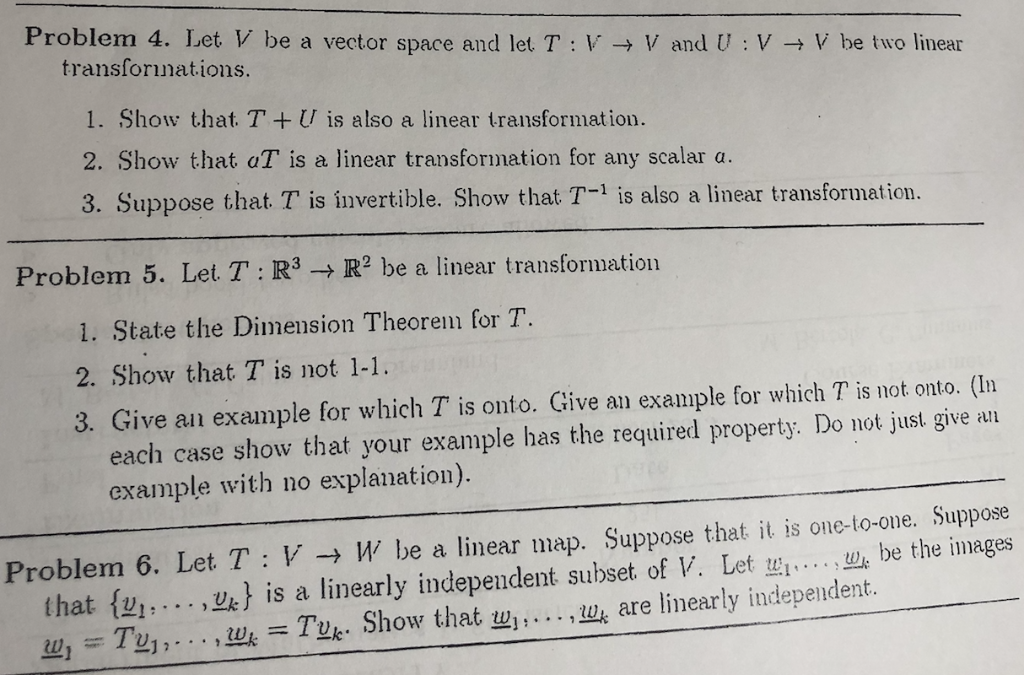Problem 4. Let V be a vector space and let T : V → V and U : V → V be two linear transforinations 1. Show that. TU is also a linear transformation. 2. Show that aT is a linear transformation for any scalar a. 3. Suppose that T is invertible. Show that T-1 is also a linear transformation. Problem 5. Let T : R3 → R2 be a linear transformatio! 1. State the Dimension Theorem for T. 2. Show that T is not 1-1. 3. Give an example for which T is onto. Give an example for which T is not onto. (In each case show that your example has the required property. Do not just give an example with no explanation). Problem 6, Let T : V → W be a linear map. Suppose that it is one-to-one. Suppose th be the images . . . . ,蚴is a linearly independent subset of V. Let wi 2], . . . ,we-Tuk. Show that w].. that { uh are linearly independent.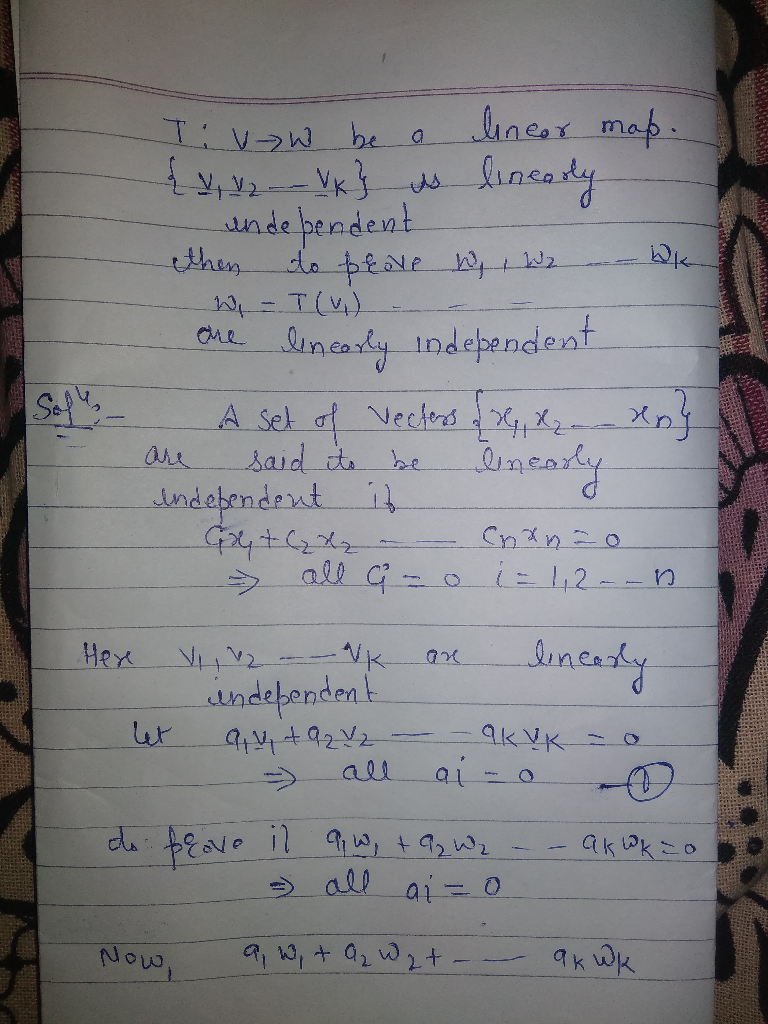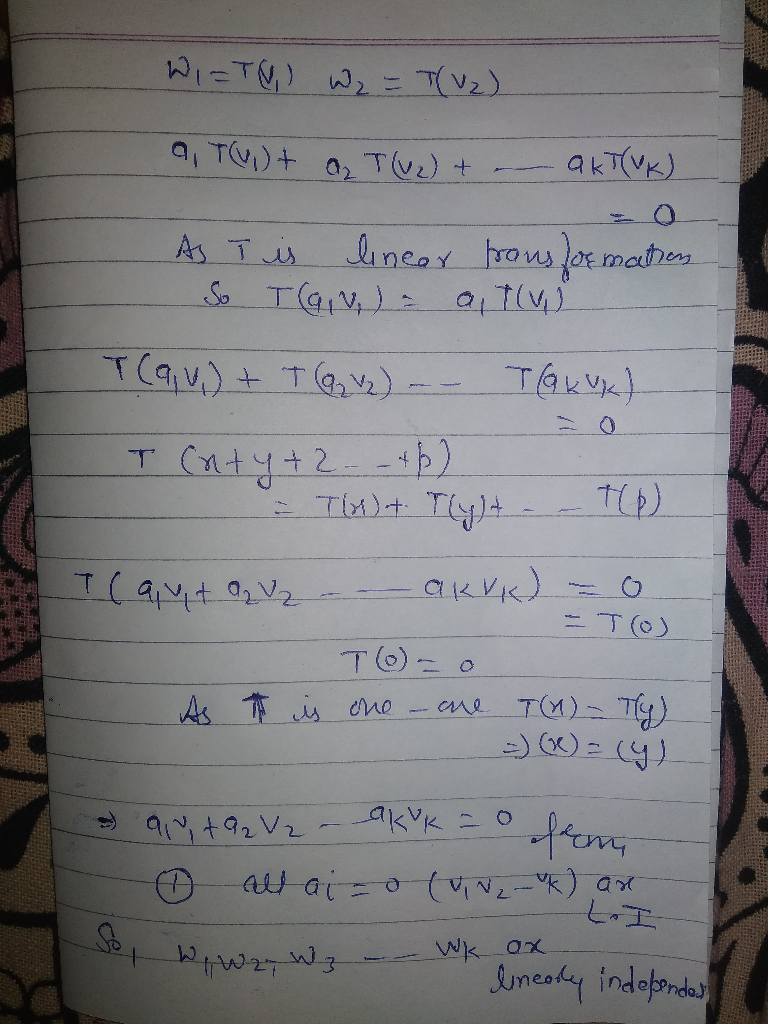##### Add Answer of: Problem 4. Let V be a vector space and let T : V → V and U : V → V be two linear transforinations...
Similar Homework Help Questions
• ### I need the answer to problem 4 (exercises 1, 2, 3) Clear and step by step...I need the answer to problem 4 (exercises 1, 2, 3) Clear and step by step please Problem 4. Let V be a vector space and let T : V → V and U : V → V be two linear transforinations 1. Show that. TU is also a linear transformation. 2. Show that aT is a linear transformation for any scalar a. 3. Suppose that T is invertible. Show that T-1 is also a linear transformation. Problem 5. Let...

• ### Please give answer with the details. Thanks a lot! Let T: V-W be a linear transformation between vector spaces V and W...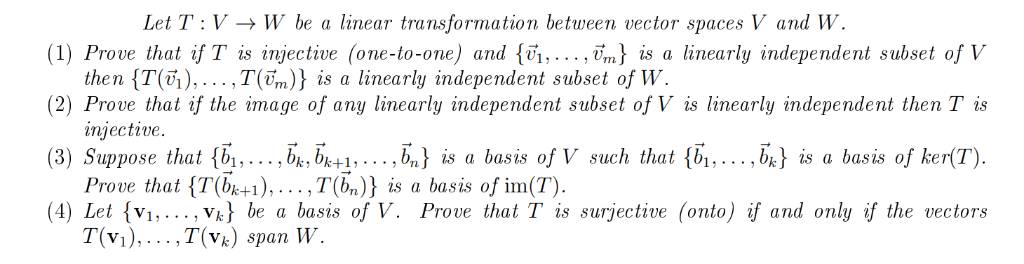Please give answer with the details. Thanks a lot! Let T: V-W be a linear transformation between vector spaces V and W (1) Prove that if T is injective (one-to-one) and {vi,.. ., vm) is a linearly independent subset of V the n {T(6),…,T(ền)} is a linearly independent subset of W (2) Prove that if the image of any linearly independent subset of V is linearly independent then Tis injective. (3) Suppose that {b1,... bkbk+1,. . . ,b,) is a...

• ### Problem 1: Let W = {p(t) € Pz : p'le) = 0}. We know from Problem...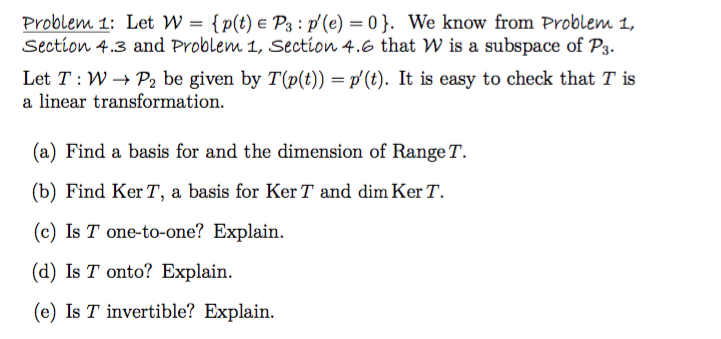Problem 1: Let W = {p(t) € Pz : p'le) = 0}. We know from Problem 1, Section 4.3 and Problem 1, Section 4.6 that W is a subspace of P3. Let T:W+Pbe given by T(p(t)) = p' (t). It is easy to check that T is a linear transformation. (a) Find a basis for and the dimension of Range T. (b) Find Ker T, a basis for Ker T and dim KerT. (c) Is T one-to-one? Explain. (d) Is...

• ### 7. Let T : V → W be a linear transformation, and let v1,v2,...,vn be vectors...

7. Let T : V → W be a linear transformation, and let v1,v2,...,vn be vectors in V. Suppose that T (v1), T (v2), . . . , T (vn) are linearly independent. Show that v1, v2, . . . , vn are linearly independent.

• ### Problem 1. The figure below shows the vectors u, v, and w, along with the images...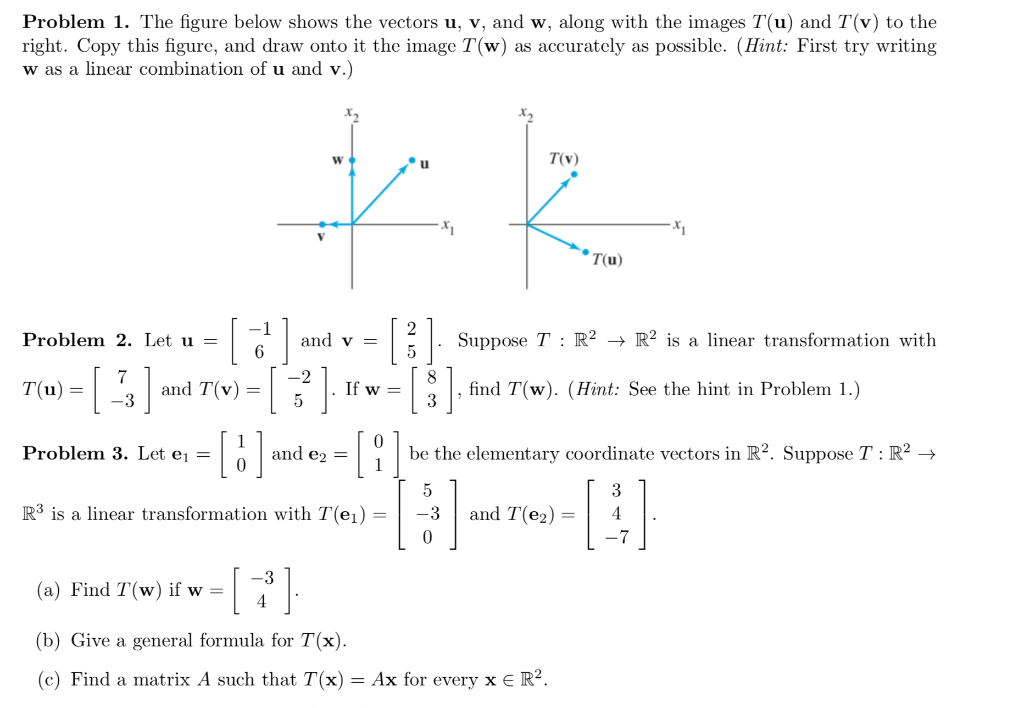Problem 1. The figure below shows the vectors u, v, and w, along with the images T(u) and T(v) to the right. Copy this figure, and draw onto it the image T(w) as accurately as possible. (Hint: First try writing w as a linear combination of u and v.) TV (u) Problem 2. Let u = | and v Suppose T : R2 + R2 is a linear transformation with 6 1 3) Tu = T(u) = -3 and T(v)...

• ### Let and let T : R4 → R4 be the map T(v) = v-2 proju,V-2 proju, w (a) Show that T is a linear tran...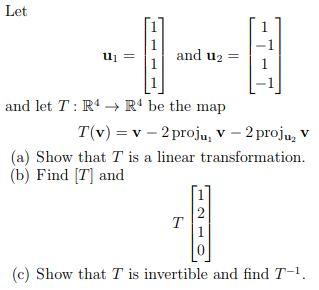Let and let T : R4 → R4 be the map T(v) = v-2 proju,V-2 proju, w (a) Show that T is a linear transformation. (b) Find T] and (c) Show that T is invertible and find T-1 Let and let T : R4 → R4 be the map T(v) = v-2 proju,V-2 proju, w (a) Show that T is a linear transformation. (b) Find T] and (c) Show that T is invertible and find T-1

• ### W is a rele that A linear transformation T from a vector space V into a...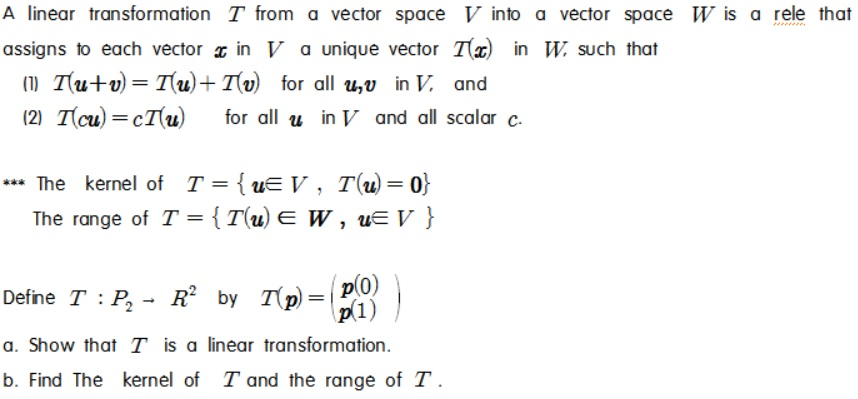W is a rele that A linear transformation T from a vector space V into a vector space assigns to each vector 2 in V a unique vector T() in W. such that (1) Tutu = Tu+Tv for all uv in V, and (2) Tſcu)=cT(u) for all u in V and all scalar c. *** The kernel of T = {UE V , T(U)=0} The range of T = {T(U) EW , ue V } Define T :P, - R...

• ### Linear Algebra-linear independence in vector spaces proofLet V be a vector space over the field F and let {v1, v2,....vk} V be linearly independent. If avectoru V is a linear combination ofv1, v2, ....vk, and w V is NOT a linear combination ofthe set{v1, v2, ....vk, tu +w} is linearly independent for any scalar tF.

• ### Prob le m 5 (Bonus 2 points) Let V be a finite dimensional vector space. Suppose that T : V -» V is matrix representati...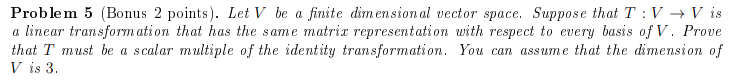Prob le m 5 (Bonus 2 points) Let V be a finite dimensional vector space. Suppose that T : V -» V is matrix representation with respect to every basis of V. Prove that the dimension of linear transform ation that has the same that T must be a scalar multiple of the identity transformation. You can assume V is 3 Prob le m 5 (Bonus 2 points) Let V be a finite dimensional vector space. Suppose that T :...

• ### 7. Let T:V : - W be a linear transformation, and let vi, U2,..., Un be...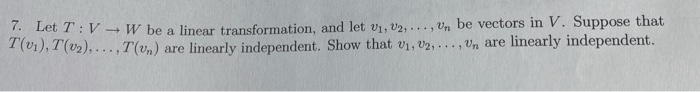7. Let T:V : - W be a linear transformation, and let vi, U2,..., Un be vectors in V. Suppose that T(01), T (v2),..., 1 (un) are linearly independent. Show that 01, V2, ..., Un are linearly independent.

Free Homework App